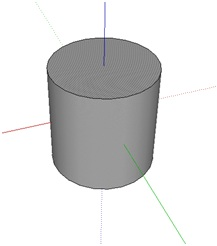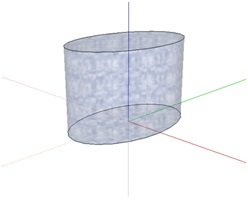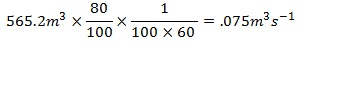# How to Find the Volume of a Cylinder

## Cylinder – Definition

Cylinder is one of the basic conic shapes found in geometry, and its properties have been known for thousands of years. In general a cylinder is defined as the set of points that lies at a constant distance from a line segment, where the line segment is known as the axis of the cylinder.

In a broader sense, a cylinder can be defined as a curved surface formed by a line segment parallel to another line segment, when travelling in a path defined by some geometrical equation. This definition allows the inclusion of several other types of cylinders into to create a cylinder family. If the cross section is an ellipse, the cylinder is an elliptical cylinder. If the cross section is a parabola or a hyperbola,it is referred to as parabolic and hyperbolic cylinders respectively.A circular cylinder can be considered as a limiting case of the n sided prisms, where n reaches infinity.

In general, the fixed line described above serves as the axis of the cylinder and either of the planar surfaces is referred to as bases. The perpendicular distance between the bases is known as the height of the cylinder.

## Using the Formula to Find the Volume of a CylinderFor a general cylinder with a base area A and height h, the volume of the cylinder is given by the formula:

Vcylinder=Ah

If the cylinder has circular cross section, the equation reduces to

V=πr2 h

where r is the radius. Even if the shapes of the cylinders are not regular, i.e. cylinders bases are not forming right angles with the curved surface, the above equations hold.

To find  the volume of a cylinder, one should know two things,

• Height of the cylinder
• The area of the cross-section – If the cylinder has circular cross section, the radius has to be known. To determine the area of elliptical or parabolic or hyperbolic, other information is needed to determine the area, and further calculation has to be carried out.

## Calculating the Volume of a Cylinder – Examples

• The inner radius of a cylindrical water tank is 3m. If the water is filled to a height of 1.5m find the volume of water included in the tank.

The radius of the base is given as 3m and the height as 1.5m. Therefore, applying the volume of a cylinder formula, we can get the volume of water in the tank.

V=πr2 h=3.14×32×1.5=42.39m3

• A cylindrical fuel tank has a diameter of 6m and a length of 20m fuel, the tank is filled only 80% of its capacity. If a motor empties the tank in 1 hour and 40 minutes, find the average volume transfer rate of the pump.

To find the volume transfer rate of the pump, the total volume pumped out has to be determined. Therefore, it is needed to calculate the volume of the tank. Since the diameter is given, we can determine the radius by formula D=2r. The radius is 3m. Using the volume of a cylinder formula we have

V=πr2 h=3.14×32×20=565.2m3

Volume of the fuel inside is only 80 of the total volume and it has taken 100 minutes to empty the tank, the volume flow rate is